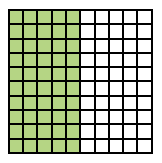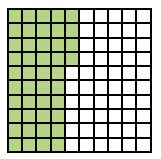### Home > ACC6 > Chapter 9 Unit 9 > Lesson CC1: 9.2.3 > Problem9-66

9-66.

Wendy and Peter each made up a new “Guess My Decimal” game just for you. Use their clues to determine the number.

1. Wendy gives you this clue: “My decimal is seven tenths greater than $\mathit{46\%}$. What is my decimal?” Show your work.

Seven tenths can also be written as $0.7$. And $46\%$ can also be written as $0.46$.

2. Peter continues the game with the following clue: “My decimal is six hundredths less than five tenths.” Use pictures and/or words to show your thinking.

• Start with five tenths. A 10 by 10 block of 100 where 5 columns out of 10 columns are shaded.

• Take six hundredths away. Changes: 6 squares, in column 5, are no longer shaded.

$\frac{5}{10} - 0.06 = 0.5 - 0.06 = 0.44$